Publicité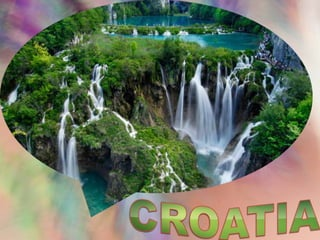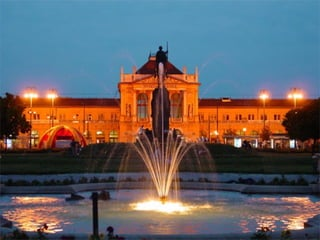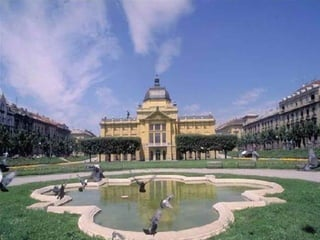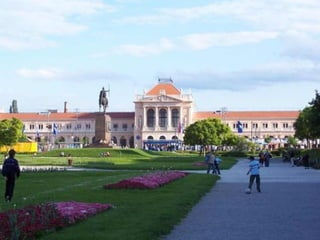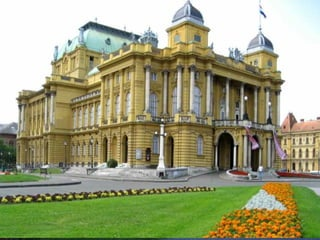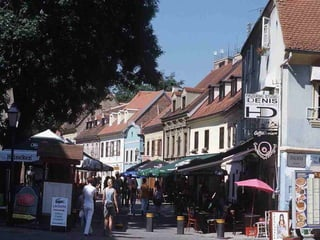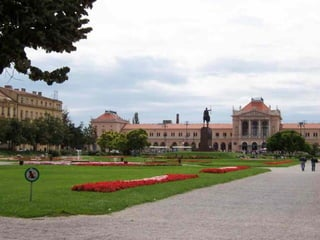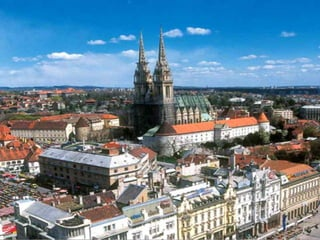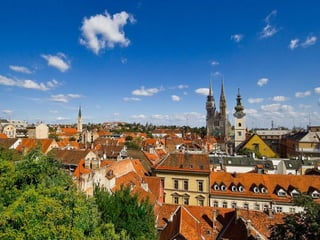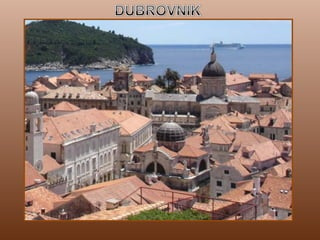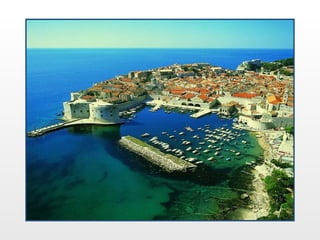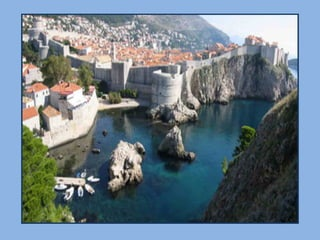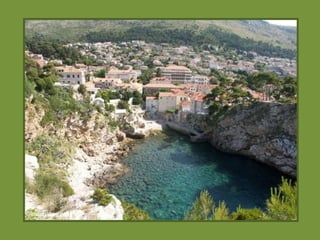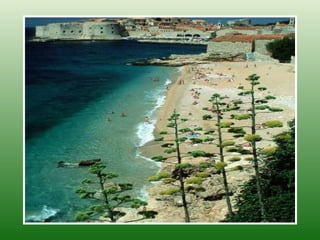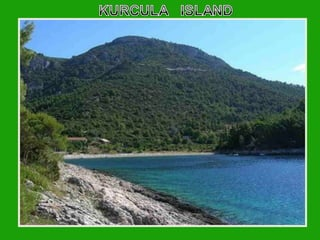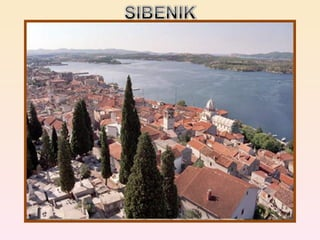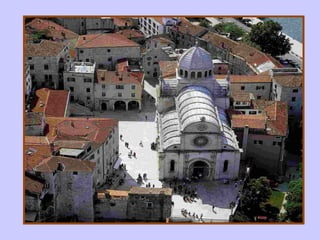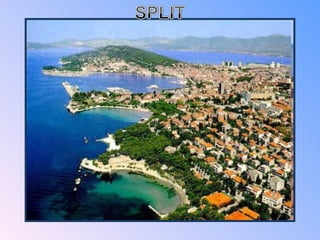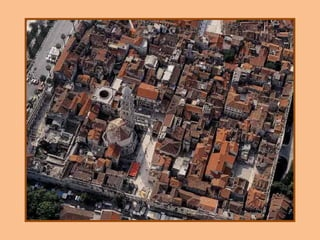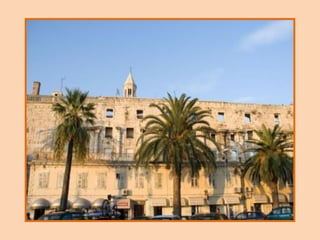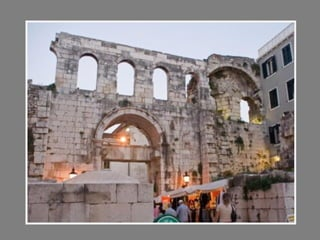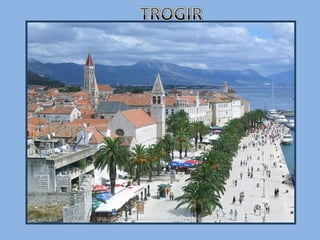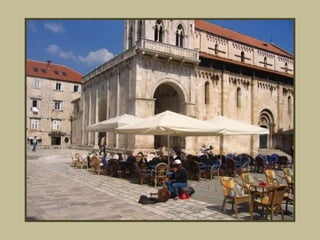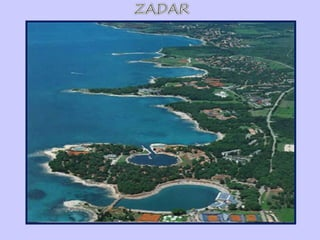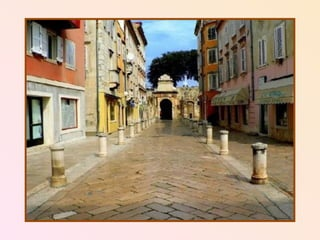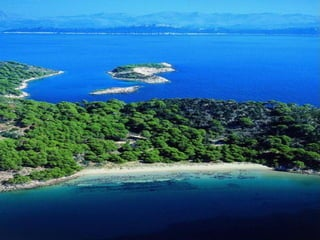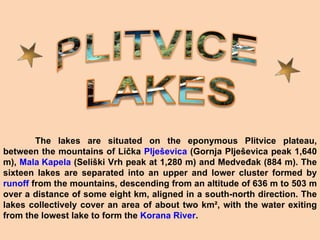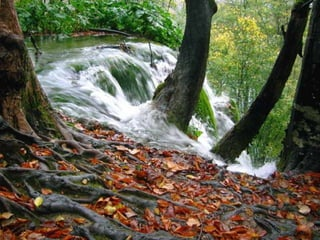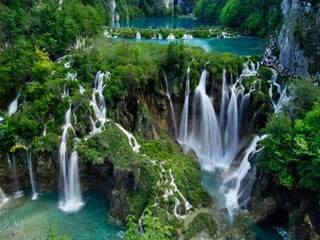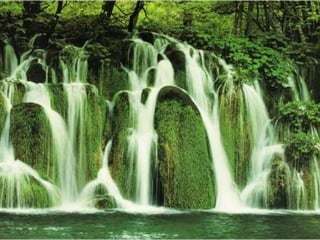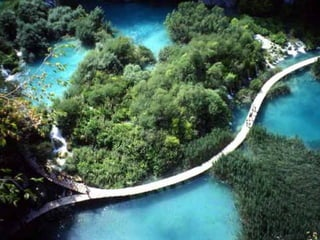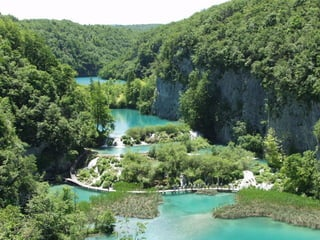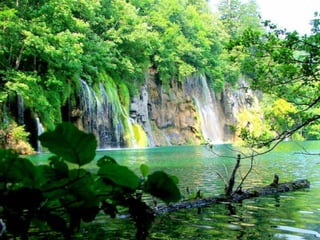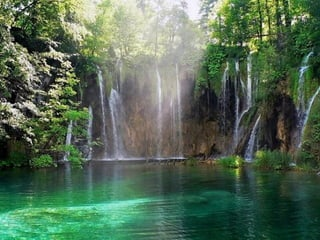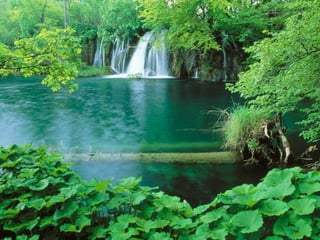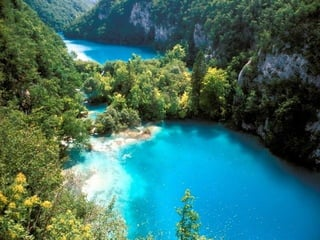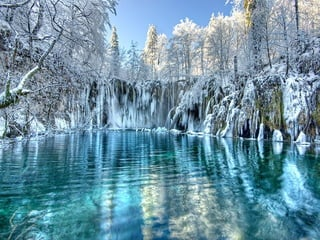1 sur 38
Publicité

### Images From Croatia

1.
2.
3.
4.
5.
6.
7.
8.
9.
10.
11.
12.
13.
14.
15.
16.
17.
18.
19.
20.
21.
22.
23.
24.
25.
26.
27.
28. The lakes are situated on the eponymous Plitvice plateau, between the mountains of Lička Plješevica (Gornja Plješevica peak 1,640 m), Mala Kapela (Seliški Vrh peak at 1,280 m) and Medveđak (884 m). The sixteen lakes are separated into an upper and lower cluster formed by runoff from the mountains, descending from an altitude of 636 m to 503 m over a distance of some eight km, aligned in a south-north direction. The lakes collectively cover an area of about two km², with the water exiting from the lowest lake to form the Korana River .
29.
30.
31.
32.
33.
34.
35.
36.
37.
38.

1. <number>
2. <number>
3. <number>
4. <number>
5. <number>
6. <number>
7. <number>
8. <number>
9. <number>
10. <number>
11. <number>
12. <number>
13. <number>
14. <number>
15. <number>
16. <number>
17. <number>
18. <number>
19. <number>
20. <number>
21. <number>
22. <number>
23. <number>
24. <number>
25. <number>
26. <number>
27. <number>
28. <number>
29. <number>
30. <number>
31. <number>
32. <number>
Publicité